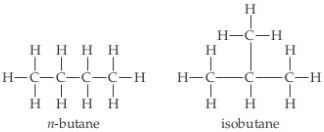# Problem: The structural formulas of the compounds n-butane and isobutane are shown below.Determine the empirical formula of each.

###### FREE Expert Solution

We have to determine the empirical formula of n-butane and isobutane using the given structural formulas.

molecule formula is a chemical formula that gives the actual number of atoms of element in a compound.

Empirical formula is a chemical formula that gives the relative proportions of elements in a compound but not the actual number of atoms of elements in a compound.

82% (447 ratings)###### Problem Details

The structural formulas of the compounds n-butane and isobutane are shown below.Determine the empirical formula of each.